CREST IQ Challenge Class 8 Sample Papers

REGISTER NOW

Syllabus:

The IQ Challenge will evaluate students on the following skills:

• Analytical reasoning
• Calculation skills
• Creative thinking
• Information processing
• Lateral thinking
• Observation skills

The questions will be a mix of Basic, Intermediate and Advanced Level.

 Q.1 Select the number which can be placed at the sign of the question mark (?) from the given alternatives.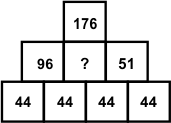Q.2 How many triangles are there in the following figure?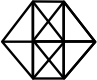Q.3 Count the number of squares in the given figure.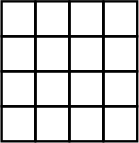Q.4 (i) Hinda, Ira, Jimmy, Kiley, Linda, Mia and Nia are sitting around a circle and are facing the centre.(ii) Nia is second to the left of Jimmy, who is to the immediate left of Mia.(iii) Hinda is third to the left of Linda.(iv) Ira is between Kiley and Linda.How many people are between Kiley and Mia?
 Q.5 Select the number that can replace the question mark ‘?’ in the following series:2, 8, 4, 64,7, 343,11, 1331, 16, ?
 Q.6 Which two signs and two numbers should be interchanged in the following equation to make it correct?(11 + 5 – 120 ÷ 4) × 20 = 40
 Q.7 Read the following diagram carefully and answer the question:Who among the following is only a graduate?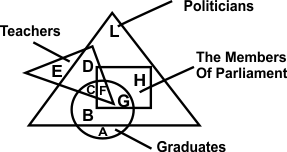Q.8 If & denotes subtraction and # denotes addition, then find the value of:15 # 65 # 95 & 47 & 74 & 32 # 51 # 74
 Q.9 If D = 4 and DRAW = 1, then how will you code LOVER?
 Q.10 Find the missing number.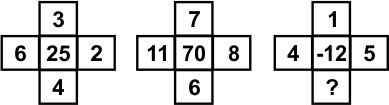Sample PDF of CREST IQ Challenge for Class 8: